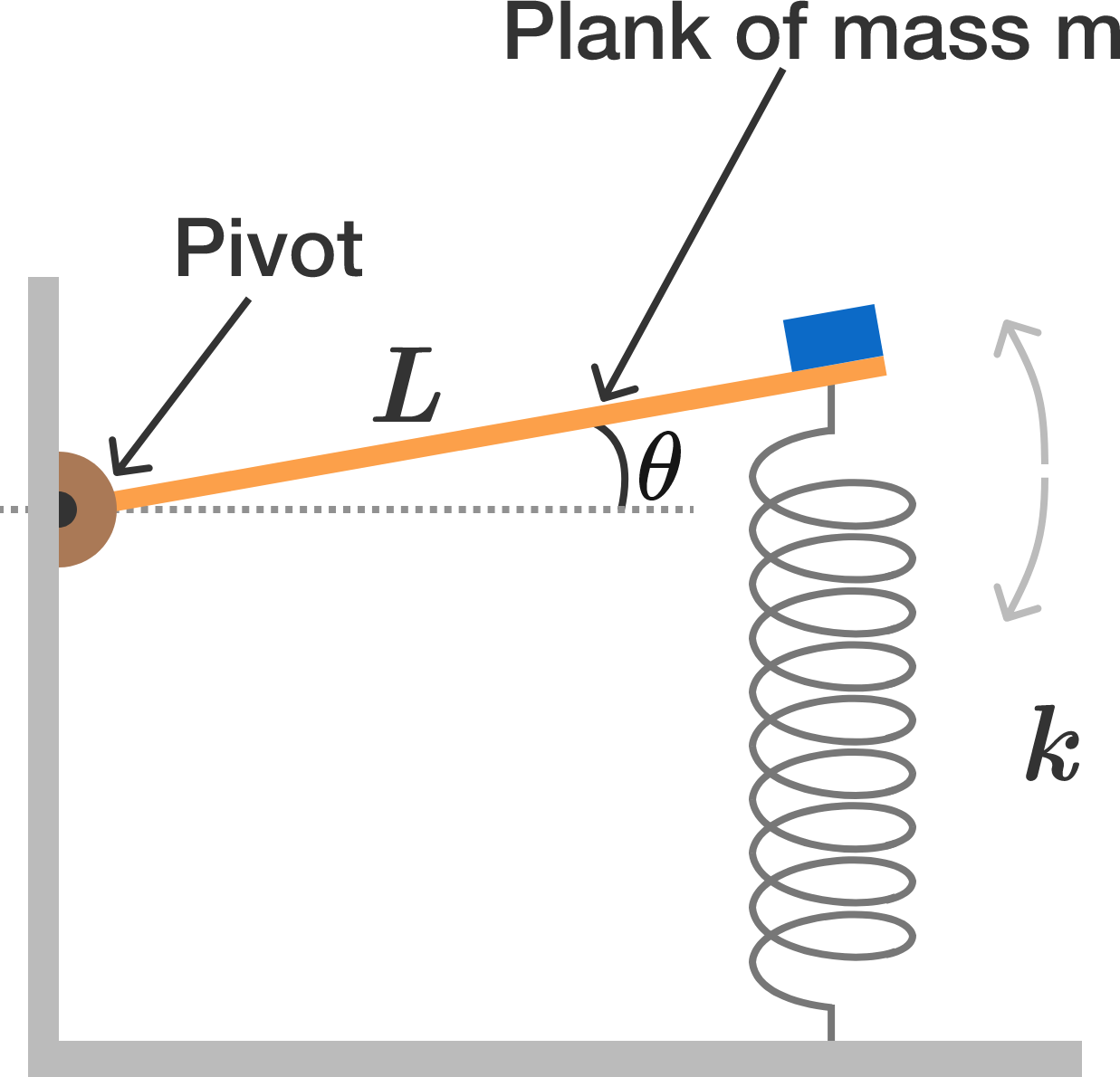# Pivoting planks.A horizontal plank of mass $m$ and length $L$ is pivoted at one end and is attached by a spring of force constant $k$ at the other end.

A small mass M is glued at the free end of the plank supported by the spring.

When the plank is displaced upward by a small angle of $\theta$ from its equilibrium position and released, it undergoes simple harmonic oscillations at angular frequency expressed as $\omega = \sqrt{\dfrac{ak}{m + bM}}.$ What is $a + b?$

×